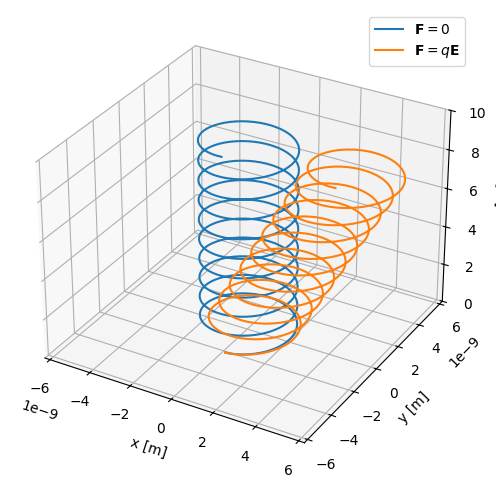# Particle drifts (plasmapy.formulary.drifts)

Functions for calculating particle drifts.

## Functions

 diamagnetic_drift(dp, B, n, q) Calculate the diamagnetic fluid perpendicular drift. ExB_drift(E, B) Calculate the "electric cross magnetic" particle drift. force_drift(F, B, q) Calculate the general force drift for a particle in a magnetic field.

## Aliases

PlasmaPy provides short-named (alias) versions of the most common plasma functionality. These aliases are only given to functionality where there is a common lexicon in the community, for example has the alias . All aliases in PlasmaPy are denoted with a trailing underscore _.

 vd_(dp, B, n, q) Alias to diamagnetic_drift. veb_(E, B) Alias to ExB_drift. vfd_(F, B, q) Alias to force_drift.

## Examples